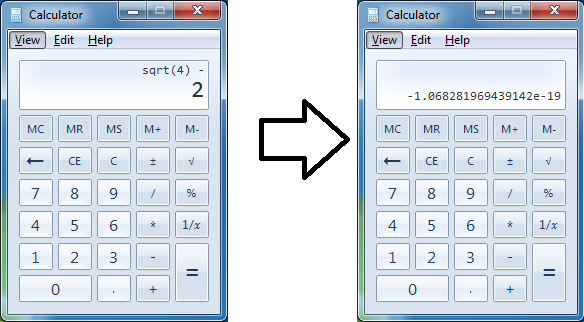# Windows Calculator

Logic Level 1I tried to find the value of $(\sqrt 4 - 2)$ in my Windows calculator, and concluded that $4 < 4$. In which of these steps did I make a flaw in my logic?

Step 1: The windows calculator evaluates that $\sqrt{4} - 2 = -1.068281969439142 \times 10^{-19}$ which is less than $0$. So we can conclude that:

$\sqrt4 - 2 < 0 .$

Step 2: Add $2$ to both sides of the inequality to obtain:

$\sqrt{4} < 2 .$

Step 3: Since both terms are positive, we can square both sides to obtain:

$4 < 4$

In which of these step did I make a flaw in my logic?

×